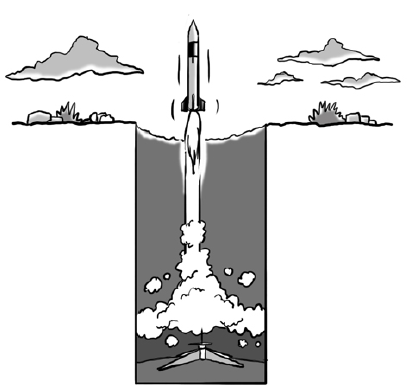### Home > INT3 > Chapter 12 > Lesson 12.1.1 > Problem12-18

12-18.

A small rocket is launched from five meters below ground level and reaches a height of $3$ meters above the ground after $4$ seconds. On the way down it is $3$ meters above the ground after $8$ seconds.

1. What are three data points that can represent this situation?

Each point is (time, height). The first one is $\left(0, −5\right)$.

2. Sketch a graph of the height of the rocket over time.

Plot the three points you listed in part (a). Then sketch a parabola through them.

3. Model the data with a quadratic function.

Substitute each point into the equation $y = ax^{2} + bx + c$.
You will have a system of three equations with three unknowns.

4. When will the rocket hit the ground?

Using your equation from part (c), let $y = 0$ and solve for $x$.
Note: You should get two answers. Which one is it?

5. What is an appropriate domain for this situation?

Look at your graph and the answer to part (d). $0\le x\le?$

6. When is the rocket below ground?

Look at your graph and the answer in part (d) that you did not use. $0\le x\le?$